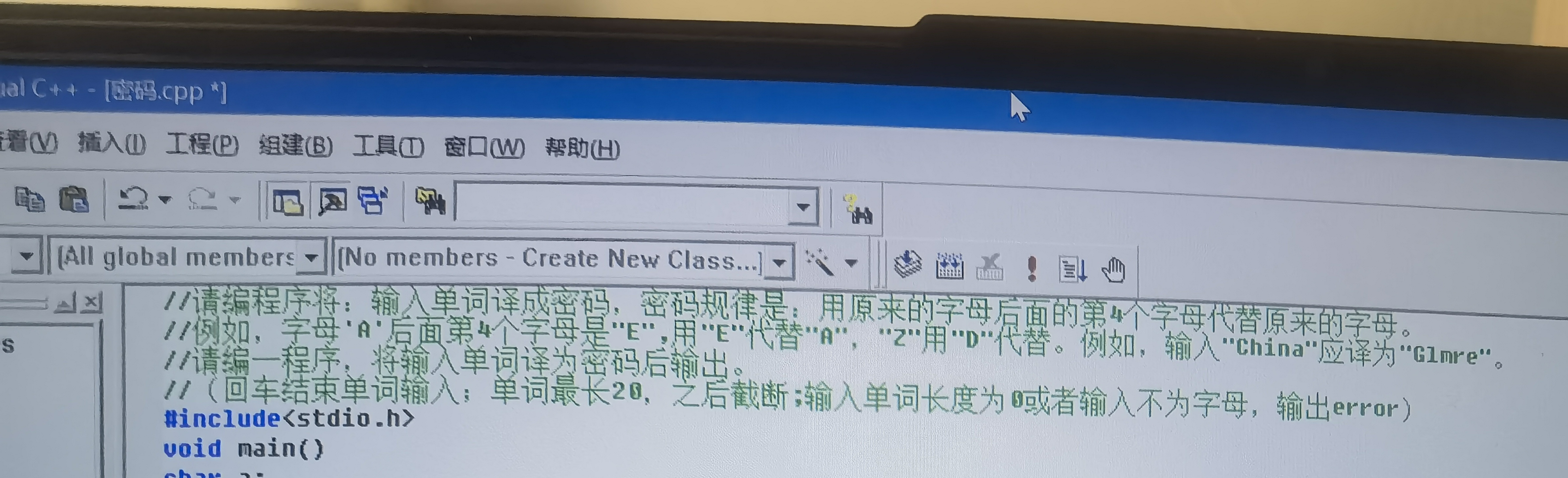2021-10-25 17:18

请问这个用c语言怎么做？• 好问题 提建议
• 收藏

1条回答默认 最新

•赵4老师 2021-10-25 18:20
已采纳

不一定对，仅供参考：

#include <ctype.h>
#include <stdio.h>
int main() {
char s;
int i;
fgets(s,21,stdin);
s=0;
for (i=0;i<20;i++)  {
if (s[i]==0 || s[i]=='\n') break;
if (!isalpha(s[i]) {
printf("error");
return 1;
}
}
for (i=0;i<20;i++)  {
if (s[i]==0 || s[i]=='\n') break;
if (s[i]<='v' && s[i]>='a' || s[i]<='V') s[i]=s[i]+4;
else if (s[i]>'V' && s[i]<'a') s[i]=s[i]-1-'V'+'A';
else s[i]=s[i]-1-'v'+'a';
}
printf("%s",s);
return 0;
}

评论
解决 无用
打赏 举报